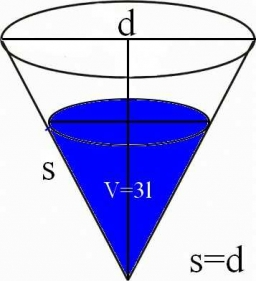# Calculate 32321

The shell of the cone is 62.8 cm2. Calculate the length of the side and height of this cone if the diameter of the base is 8 cm.

s =  5 cm
h =  3 cm

### Step-by-step explanation:Did you find an error or inaccuracy? Feel free to write us. Thank you!

Tips for related online calculators
Need help calculating sum, simplifying, or multiplying fractions? Try our fraction calculator.
The Pythagorean theorem is the base for the right triangle calculator.

#### You need to know the following knowledge to solve this word math problem:

We encourage you to watch this tutorial video on this math problem: